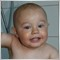# RSI indicator - page 629505

drunkpips:
3 different rsi crossing each other and also crossing zero

Drunkpips, just guessing you meant crossing 50, that's how i made this one.

Files:
rsi_x3.png 51 kb9505

winner246:

Please kindly assist with the HISTO VERSION of these 3 indicators based ON THEIR GREEN AND RED COLOR SIGNALS ONLY.

Histo version of Rmi rsx smoothed here https://www.mql5.com/en/forum/183640346

thanks mrtools ,

how about an arrow whenever the 2 smaller period rsi cross the big period e.g

an arrow when rsi 7 and 14 are both above or below rsi 21

the second arrow will be when the 3 rsi cross 50 to either the down or up side

TIA181920

drunkpips:
thanks mrtools ,

how about an arrow whenever the 2 smaller period rsi cross the big period e.g

an arrow when rsi 7 and 14 are both above or below rsi 21

the second arrow will be when the 3 rsi cross 50 to either the down or up side

TIA

drunkpips

Case when 2 values are crossing the third value at a same time is rather an exception than a rule. Much more probable is that you will get the state you are looking for when the 2 slowest rsi cross each other330

Hello mrtools,

thank you very much for the histo version of rmi rsx smoothed.346

drunkpips Case when 2 values are crossing the third value at a same time is rather an exception than a rule. Much more probable is that you will get the state you are looking for when the 2 slowest rsi cross each other

i really need a signal only when all 3 rsi are either above or below zero. it shouldnt matter whether the 2 smaller rsi are above or below the larger rsi.

can you help me181920

drunkpips:
i really need a signal only when all 3 rsi are either above or below zero. it shouldnt matter whether the 2 smaller rsi are above or below the larger rsi. can you help me

drunkpips

You mean above or bellow 50?181920

Been fooling with a function to calculate double smoothed stochastic of any value

First choice of the "any value" was rsi. Somewhat surprising calculation a double smoothed stochastic compared to the same double smoothed stochastic of pure prices does not lag. Quite the contrary : in some cases it is significantly faster (see the last part of the example picture : upper is the dss of rsi lower is the dss of a price with exactly the same settings). So, it seems, that sometimes it using an additional step does not necessarily mean a lag

Files:
dss.gif 40 kb346

drunkpips You mean above or bellow 50?

yes boss, i mean whenever all 3 rsi are either above or below 5054

mrtools:
Well, I hope you get my point